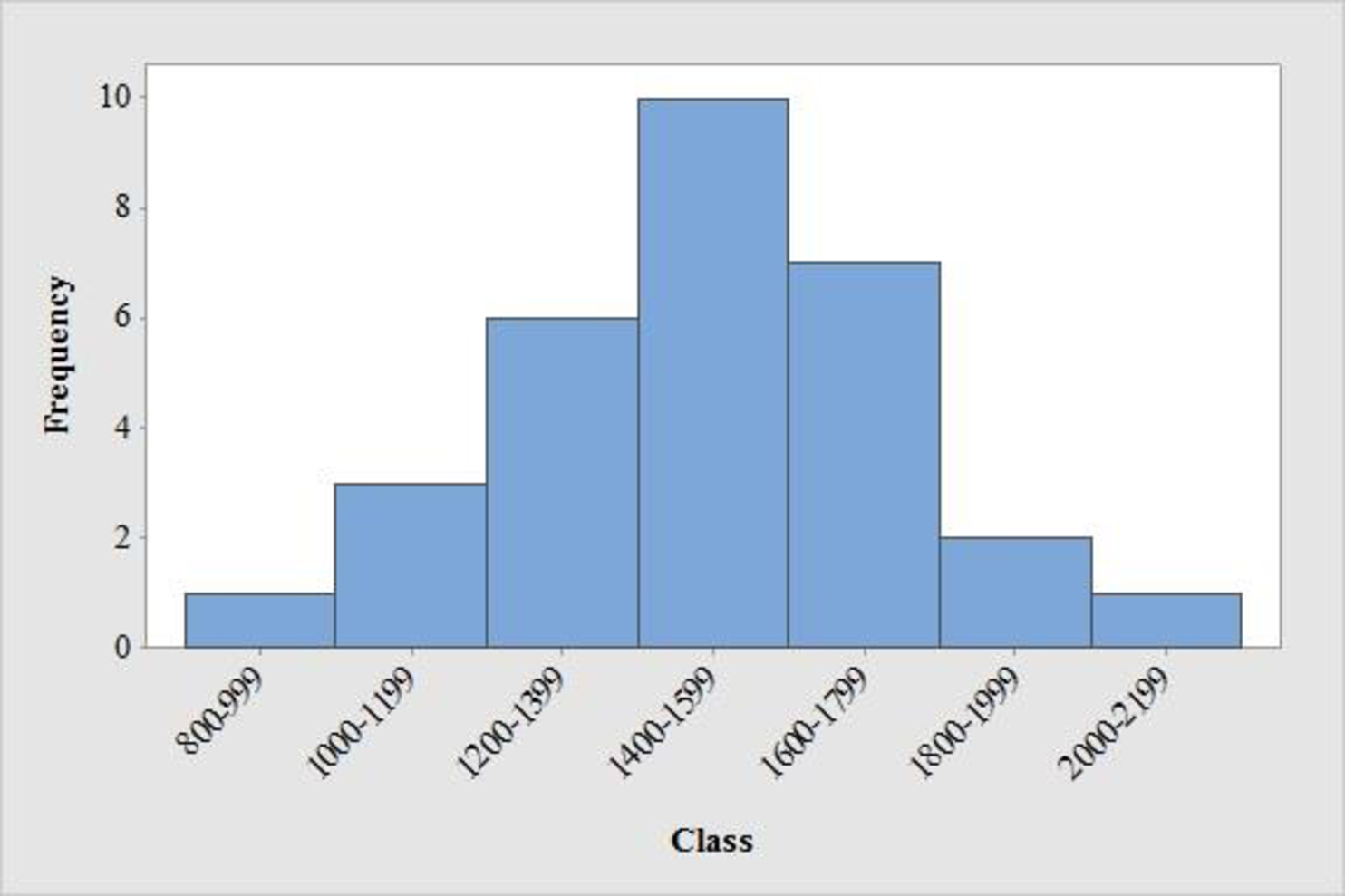# Construct the frequency distribution and histogram for the data.13th Edition
Anderson
Publisher: CENGAGE L
ISBN: 978130588188413th Edition
Anderson
Publisher: CENGAGE L
ISBN: 9781305881884

#### Solutions

Chapter 2, Problem 44SE

a.

To determine

## Construct the frequency distribution and histogram for the data.

Expert Solution

The frequency distribution is tabulated below:

 Class Tally Frequency 800-999 | 1 1000-1199 ||| 3 1200-1399 ||||| 6 1400-1599 |||||||| 10 1600-1799 |||||| 7 1800-1999 || 2 2000-2199 | 1 Total 30
• Output using MINITAB software is given below:### Explanation of Solution

Calculation:

The data represents the sample of SAT scores for combined three part sat scores.

The frequencies are calculated by using the tally mark and the method of grouping is used because, range of the data is from 800 to 2,199. Here, the number of times each class levels repeats is the frequency of that particular class.

• From the given data set it is given that the class should be from approximately started with the class of 800 with class width of about 200.
• Make a tally mark for each value in the corresponding class and continue for all values in the data.
• The number of tally marks in each class represents the frequency, f of that class.

Similarly, the frequency of remaining classes for the data set is given below:

 Class Tally Frequency 800-999 | 1 1000-1199 ||| 3 1200-1399 ||||| 6 1400-1599 |||||||| 10 1600-1799 |||||| 7 1800-1999 || 2 2000-2199 | 1 Total 30

Software procedure:

Step by step procedure to draw the frequency histogram chart by using MINITAB software.

• Choose Graph > Histogram.
• Choose Simple, and then click OK.
• In Graph variables, enter the corresponding column of data.
• In scale on y-axis, make click on frequency.
• Click on ok.

b.

To determine

### Comment the shape of the distribution.

Expert Solution

The distribution of sat score is approximately symmetric.

### Explanation of Solution

Symmetric distribution:

When the left and right sides of the distribution are approximately equal or mirror images of each other and three distributions will fall under symmetric distribution that is bell shaped, triangular and uniform or rectangular and then it is symmetric distribution.

From the graph, it is observed that the left and right side of the histogram is approximately equal. Hence, the shape of the distribution is bell-shaped curve.

Thus, it can be concluded that the distribution is approximately symmetric.

c.

To determine

Expert Solution

### Explanation of Solution

From the data and the histogram, it is observed that approximately 33 percent of sat score (frequency about 10 of 30) will lie between 1400 and 1599.

• Moreover, the score less than 800 or greater than 2,200 are unusual and the average value of sat scores is over 1,500.

### Want to see more full solutions like this?

Subscribe now to access step-by-step solutions to millions of textbook problems written by subject matter experts!

### Want to see more full solutions like this?

Subscribe now to access step-by-step solutions to millions of textbook problems written by subject matter experts!